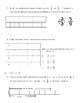# Fractions TestSubject
Resource Type
File Type

Word Document File

(101 KB|7 pages)
Product Rating
Standards
• Product Description
• StandardsNEW

This was created to be a end fractions unit test for 4th grade. It covers equivalent fractions, comparing fractions, decomposing fractions, addition & subtractions of fractions and fraction models. I encourage you to use the word format and change the names in the questions to your name and the the names of students in your classes (they love that)!

Solve word problems involving addition and subtraction of fractions referring to the same whole and having like denominators, e.g., by using visual fraction models and equations to represent the problem.
Decompose a fraction into a sum of fractions with the same denominator in more than one way, recording each decomposition by an equation. Justify decompositions, e.g., by using a visual fraction model. Examples: 3/8 = 1/8 + 1/8 + 1/8; 3/8 = 1/8 + 2/8; 2 1/8 = 1 + 1 + 1/8 = 8/8 + 8/8 + 1/8.
Understand addition and subtraction of fractions as joining and separating parts referring to the same whole.
Understand a fraction 𝘢/𝘣 with 𝘢 > 1 as a sum of fractions 1/𝘣.
Compare two fractions with different numerators and different denominators, e.g., by creating common denominators or numerators, or by comparing to a benchmark fraction such as 1/2. Recognize that comparisons are valid only when the two fractions refer to the same whole. Record the results of comparisons with symbols >, =, or <, and justify the conclusions, e.g., by using a visual fraction model.
Total Pages
7 pages
Not Included
Teaching Duration
N/A
Report this Resource to TpT
Reported resources will be reviewed by our team. Report this resource to let us know if this resource violates TpT’s content guidelines.